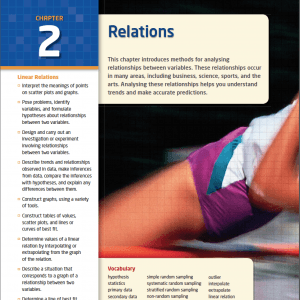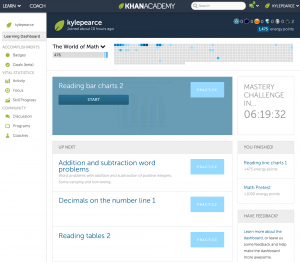Many of these resources were created and/or modified by Dave Bracken with further additions and modifications by Kyle Pearce.The MPM1D Grade 9 Academic mathematics course enables students to develop an understanding of mathematical concepts related to algebra, analytic geometry, and measurement and geometry through investigation, the effective use of technology, and abstract reasoning. Students will investigate relationships, which they will then generalize as equations of lines, and will determine the connections between different representations of a linear relation. They will also explore relationships that emerge from the measurement of three-dimensional figures and two-dimensional shapes. Students will reason mathematically and communicate their thinking as they solve multi-step problems. Click to view MPM1D Strands and Overall Expectations.

 MPM1D – Grade 9 Academic – Difficulty With Homework Form This link will allow you indicate which question you would like to look at from the homework. Be sure to take a minute to think about which question gave you the most trouble.

## MPM1D Digital Textbook

### McGraw-Hill Ryerson: Principles of Mathematics, 9While we don’t use the textbook for much more than a question bank, you can access the McGraw-Hill Ryerson Textbook: Principles of Mathematics, 9 Electronic Textbook in PDF Form. Note that the textbook is password protected for only my students due to copyright law.Access 3 Act Math Tasks related to the MPM1D Grade 9 Academic Math Course.Access Khan Academy Practice Exercise Links organized by MPM1D Grade 9 Academic Math Topics to allow you to deepen your understanding of the overall and specific expectations in this course.

## MPM1D Grade 9 Academic Topics of Study: Course Notes & Note Solutions

#### MPM1D Unit 1 (Unit 2 in Textbook) – Relations

 Sec. 1.1 (2.3 in Text) – Use Scatter Plots to Analyse Data Blank Note Handout 1, Handout 2 Note Solutions 1, Solutions 2 Corresponding Textbook Pages: 56 to 67 Sec. 1.2 (2.4 in Text) – Trends, Interpolation, and Extrapolation Blank Note Handout Note Solutions Corresponding Textbook Pages: 68 to 76 Sec. 1.3 (2.5 in Text) – Linear and Non-Linear Relations Blank Note Handout Note Solutions Corresponding Textbook Pages 77 to 87 Sec. 1.4 (2.6 in Text) – Distance-Time Graphs (Part 1) Blank Note Handout Note Solutions Corresponding Textbook Pages 88 to 94 Sec. 1.5 (2.6 in Text) – Distance-Time Graphs (Part 2) Blank Note Handout Part 1, Handout Part 2 Handout 1 Solutions, Handout 2 Solutions Corresponding Textbook Pages 88 to 94 Sec. 1.6 (2.7 in Text) – Unit 2 Review Blank Note Handout v1 Blank Note Handout v2 Note Solutions v1 Note Solutions v2 Corresponding Textbook Pages 95 to 99

### MPM1D Unit 2 (Unit 3 in Textbook) – Polynomials

 Sec. 2.1 (3.1 in Text) – Build Algebraic Models Using Concrete Materials SMART Notebook File Blank Note Handout Note Solutions Corresponding Textbook Pages 104 to 109 Sec. 2.2 (3.2 in Text) – Work With Exponents Blank Note Handout Note Solutions Corresponding Textbook Pages 110 to 118 Sec. 2.3 (3.3 in Text) – Discover the Exponent Laws (Part 1) Blank Note Handout Note Solutions Corresponding Textbook Pages 119 to 129 Sec. 2.3 (3.3 in Text) – Discover the Exponent Laws (Part 2) Blank Note Handout Note Solutions Corresponding Textbook Pages 119 to 129 Sec. 2.4 (3.4 in Text) – Communicate With Algebra (Part 1) Blank Note Handout Note Solutions Corresponding Textbook Pages 134 to 135 Sec. 2.5 (3.5 in Text) – Collect Like Terms Blank Note Handout Note Solutions Corresponding Textbook Pages 144 to 153 Sec. 2.6 (3.6 in Text) – Add and Subtract Polynomials Blank Note Handout Note Solutions Corresponding Textbook Pages 154 to 159 Sec. 2.7 (3.7 in Text) – The Distributive Property Blank Note Handout Note Solutions SMART Notebook File Corresponding Textbook Pages 160 to 173 Sec. 2.8 – Adding/Subtracting Polynomials & Distribution Assignment Blank Note Handout Sec. 2.9 (3.8 in Text) – Unit 2 Review & Practice Test Blank Note Handout Note Solutions Corresponding Textbook Pages 174 to 177 Unit 2 Recap Blank Note Handout Note Solutions

### MPM1D Unit 3 (Unit 4 in Textbook) – Equations

 Sec. 3.1 (4.1 in Text) – Solve Simple Equations Blank Note Handout Note Solutions Corresponding Textbook Pages 186 to 195 Sec. 3.2 (4.2 in Text) – Solve Multi-Step Equations Day 1 Blank Note Handout Day 1 Note Solutions Day 2 Blank Note Handout Day 2 Note Solutions Corresponding Textbook Pages 196 to 203 Sec. 3.3 (4.3 in Text) – Solve Equations Involving Fractions Day 1 Blank Note Handout Day 1 Note Solutions Day 2 Blank Note Handout Day 2 Note Solutions Corresponding Textbook Pages 204 to 210 Sec. 3.4 (4.4 in Text) – Modelling With Formulas Day 1 Blank Note Handout Day 1 Note Solutions Day 2 Blank Note Handout Day 2 Note Solutions Corresponding Textbook Pages 211 to 219 Sec. 3.5 (4.5 in Text) – Modelling With Algebra Blank Note Handout Note Solutions Corresponding Textbook Pages 220 to 229 Sec. 3.6 (4.6 in Text) – Chapter 3 Review – Equations Blank Note Handout Note Solutions Corresponding Textbook Pages 230 to 233

### MPM1D Unit 4 (Unit 5 in Textbook) – Modelling With Graphs

 Sec. 4.1 (5.1 in Text) – Direct and Partial Variation Day 1 Blank Note Handout – OLD Note Solutions – OLD NEW Blank Note Handout – Direct AND Partial Variation New Note Solutions Corresponding Textbook Pages 238 to 245 Sec. 4.2 (5.2 in Text) – Direct and Partial Variation Day 2 Blank Note Handout – OLD Note Solutions – OLD NEW Blank Note Handout – Direct AND Partial Variation New Note Solutions Corresponding Textbook Pages 246 to 253 Sec. 4.3 (5.3 in Text) – Slope Blank Note Handout Part 1 Note Solutions Part 1 Blank Note Handout Part 2 Note Solutions Part 2 Corresponding Textbook Pages 254 to 263 Sec. 4.4 (5.4 in Text) – Slope as a Rate of Change Blank Note Handout Part 1 Note Solutions Part 1 Blank Note Handout Part 2 Note Solutions Part 2 Corresponding Textbook Pages 264 to 271 Sec. 4.5 (5.5 in Text) – First Differences Blank Note Handout Note Solutions Corresponding Textbook Pages 272 to 278 Sec. 4.6 (5.6 in Text) – Connecting Variation, Slope, and First Differences Blank Note Handout Note Solutions Corresponding Textbook Pages 279 to 287 Sec. 4.7 (5.7 in Text) – Unit 4 Review – Modelling With Graphs Blank Note Handout Part 1 Note Solutions Part 1 Blank Note Handout Part 2 Corresponding Textbook Pages 279 to 287

### MPM1D Unit 5 (Unit 6 in Textbook) – Analyse Linear Relations

 Sec. 5.1 (6.1 in Text) – The Equation of a Line in Slope y-Intercept Form: y = mx + b Blank Note Handout Note Solutions Corresponding Textbook Pages 296 to 307 Sec. 5.2 (6.2 in Text) – The Equation of a Line in Standard Form: Ax + By + C = 0 Blank Note Handout Note Solutions Corresponding Textbook Pages 308 to 314 Sec. 5.3 (6.3 in Text) – Graph a Line Using Intercepts Blank Note Handout Note Solutions Corresponding Textbook Pages 315 to 325 Sec. 5.4 (6.4 in Text) – Parallel and Perpendicular Lines Blank Note Handout Note Solutions Corresponding Textbook Pages 326 to 329 Sec. 5.5 (6.5 in Text) – Find an Equation for a Line Given the Slope and a Point Blank Note Handout Note Solutions Corresponding Textbook Pages 330 to 337 Sec. 5.6 (6.6 in Text) – Find an Equation for a Line Given Two Points Blank Note Handout Note Solutions Corresponding Textbook Pages 338 to 343 Sec. 5.7 (6.7 in Text) – Linear Systems Blank Note Handout Note Solutions Corresponding Textbook Pages 344 to 351 Sec. 5.8 (6.8 in Text) – Unit 6 Review – Analyse Linear Relations Blank Note Handout Note Solutions Corresponding Textbook Pages 352 to 355

### MPM1D Unit 6 (Unit 7 in Textbook) – Geometric Relationships

 Sec. 6.1 (7.1 in Text) – Angle Relationships in Triangles Blank Note Handout Note Solutions Corresponding Textbook Pages 364 to 373 Sec. 6.2 (7.2 in Text) – Angle Relationships in Quadrilaterals Blank Note Handout Note Solutions Corresponding Textbook Pages 374 to 383 Sec. 6.3 (7.3 in Text) – Angle Relationships in Polygons Blank Note Handout Note Solutions Corresponding Textbook Pages 384 to 393 Sec. 6.4 (7.4 in Text) – Midpoints and Medians in Triangles Blank Note Handout Note Solutions Corresponding Textbook Pages 394 to 400 Sec. 6.5 (7.5 in Text) – Midpoints and Diagonals in Quadrilaterals Blank Note Handout Note Solutions Corresponding Textbook Pages 401 to 407 Sec. 6.6 (7.6 in Text) – Unit 6 Review – Geometric Relationships Blank Note Handout Note Solutions Corresponding Textbook Pages 408 to 411

### MPM1D Unit 7 (Unit 8 in Textbook) – Measurement Relationships

 Sec. 7.1 (8.1 in Text) – Apply the Pythagorean Theorem Blank Note Handout Note Solutions Corresponding Textbook Pages 418 to 425 Sec. 7.2 (8.2 in Text) – Perimeter and Area of Composite Figures Blank Note Handout Note Solutions Corresponding Textbook Pages 426 to 435 Sec. 7.3 (8.3 in Text) – Surface Area and Volume of Prisms and Pyramids Blank Note Handout Note Solutions Corresponding Textbook Pages 436 to 443 Sec. 7.4 (8.4 in Text) – Surface Area of a Cone Blank Note Handout Note Solutions Corresponding Textbook Pages 444 to 450 Sec. 7.5 (8.5 in Text) – Volume of a Cone Blank Note Handout Note Solutions Corresponding Textbook Pages 451 to 456 Sec. 7.6 (8.6 in Text) – Surface Area of a Sphere Corresponding Textbook Pages 457 to 461 Sec. 7.7 (8.7 in Text) – Volume of a Sphere Corresponding Textbook Pages 457 to 461 Sec. 7.8 (8.8 in Text) – Unit 7 Review – Measurement Relationships Corresponding Textbook Pages 470 to 473

### MPM1D Unit 8 (Unit 9 in Textbook) – Optimizing Measurements

 Sec. 8.1 (9.1 in Text) – Investigate Measurement Concepts Blank Note Handout Note Solutions Corresponding Textbook Pages 478 to 483 Sec. 8.2 (9.2 in Text) – Perimeter and Area Relationships of a Rectangle Blank Note Handout Note Solutions Corresponding Textbook Pages 484 to 490 Sec. 8.3 (9.3 in Text) – Minimize the Surface Area of a Square-Based Prism Blank Note Handout Note Solutions Corresponding Textbook Pages 491 to 497 Sec. 8.4 (9.4 in Text) – Maximize the Volume of a Square-Based Prism Corresponding Textbook Pages 498 to 503 Sec. 8.5 (9.5 in Text) – Maximize the Volume of a Cylinder Corresponding Textbook Pages 504 to 509 Sec. 8.6 (9.6 in Text) – Minimize the Surface Area of a Cylinder Corresponding Textbook Pages 510 to 515 Sec. 8.7 (9.7 in Text) – Unit 8 Review – Optimizing Measurements Corresponding Textbook Pages 516 to 519

## Strands and Overall Expectations – MPM1D Principles of Mathematics, Grade 9 Academic

### Analytic Geometry

• AG1 – Investigating the Relationship Between the Equation of a Relation and the Shape of Its Graph
• AG2 – Investigating the Properties of Slope
• AG3 – Using the Properties of Linear Relations to Solve Problems

### Measurement and Geometry